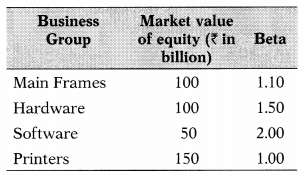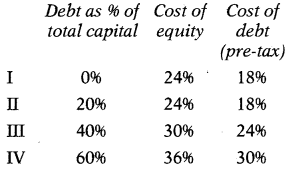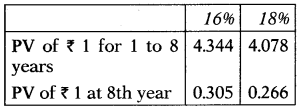# Cost of Capital – Financial and Strategic Management MCQ

0

Cost of Capital – Financial and Strategic Management MCQ

1 / 45

Ganesh Ltd. requires an amount of ₹ 5,00,000 to finance a project. It was decided to raise such finance by the issue of debentures. Cost of debt is 10% (before tax) up to ₹ 2,00,000 and 13% (before tax) beyond that. The tax rate is 30%. What is the average marginal cost of capital of new finance of ₹ 5,00,000?

2 / 45

The Company can issue a 14% new debenture. The company’s debenture is currently selling at ₹ 98. The face value of debenture is ₹ 100. The company’s marginal tax rate is 50%. What is the cost of debenture – (i) based on book value; (a) based on market value?

3 / 45

You are analyzing the beta for A Ltd. and have divided the Company into four broad business groups, with market values and betas for each 1 group.An Ltd. had Z 50 billion in debt outstanding. The market risk premium is 8.5. The Treasury bond rate is 7.5%. Estimate cost of equity for A Ltd.

4 / 45

Calculate the marginal cost of capital (MCC) for the firm if it raises ₹ 750 million for a new project. The firm plans to have a target debt to value ratio of 20%. The beta of a new project is 1.4375. The debt capital will be raised through term loans. It will carry an interest rate of 9.5% for the first ₹ 100 million and 10% for the next ₹ 50 million.The current market price per equity share is ₹ 60. The prevailing default risk-free interest rate on 10-year GOI Treasury bonds is 5.5%. The average market risk premium is 896.The current market price per equity share is ₹ 60. The prevailing default risk-free interest rate on 10-year GOI Treasury bonds is 5.5%. The average market risk premium is 896.

5 / 45

ABC Ltd. has three divisions: A, B & C. Division A has the least risk and Division C has the most risk. ABC Ltd. has an after-tax cost of debt of 4.6% and a cost of equity of 9.5%. Company is financed with 50% debt & 50% equity. Management has told the Divisional Manager of Division A that projects in that division are assigned a discount rate that is 1% less than the firm’s weighted average cost of capital. What is the discount rate applicable to Division A?

6 / 45

Baba Ltd. has a cost of equity of 12%, a pre-tax cost of debt of 7%, and a tax rate of 35%. What is the firm’s weighted average cost of capital if the debt-equity ratio is 0.60?

7 / 45

Jackson Ltd. has 12,000 bonds outstanding at a quoted price of 98% of face value. The bonds mature in 11 years and carry a 9% annual coupon. What is your best estimate of Jackson’s after-tax cost of debt if the applicable tax rate is 35%?

8 / 45

Calculate the weighted marginal cost of capital for the company, if it raises ₹ 10 Crores next year, given the following information:(i) Next expected dividend is ₹ 3.60 and expected to grow at the rate of 7%.(ii) Income-tax rate is 40%.(iii) Amount will be raised by equity and debt in equal proportions.(iv) Additional issue of equity shares will result in the next price per share being fixed at ₹ 32.(v) Debt capital raised by way of term loans will cost 15% for the first ₹ 2.5 Crores and 16% for the balance.

9 / 45

AB Ltd. estimates the cost of equity and debt components of its capital for different levels of debt; equity mix as follows:The tax rate is 50%Select the best capital mix for the company so that its overall cost of capital is minimum.

10 / 45

Debt as a percentage of the total capital of Tiger Ltd. is 60%. Its cost of equity is 24% and the pre-tax cost of debt is 20%. The tax rate is 50%. What is the overall cost of capital of Tiger Ltd.?

11 / 45

The following is the structure of a company: capitalThe current market price of equity share is ₹ 200. For the last year, the company had paid an equity dividend at 25% and itsdividend is likely to grow 5% every year. The corporate tax rate is 30%. Compute WACC on the basis of market value.

12 / 45

A Company has ₹ 180 Million of 10% Debentures having a face value of ₹ 100. The debentures are redeemable after 3 years and interest is paid annually. The current ex-interest debenture market value is ₹ 103.The pre-tax cost of debentures on the basis of the present value of future cash flow shall be –Use the following rates for your calculations:13 / 45

Compute the EVA with the help of the following information:

 ₹ Equity 10,00,000 Debt (10%) 5,00,000 Profit after tax 2,00,000Risk-free rate of return is 7%.Beta (β) = 0.9,Market rate of return = 15%.

14 / 45

Raja Ltd. has 8% Debentures (Face value ₹ 2,500) of ₹ 9,00,000 which are redeemable at 5% premium, sold at 98%, 3% flotation costs with maturity of 20 years. The corporate tax rate is 35%. The company paid debenture interest of ₹ 60,000 out of the total interest payable of ₹ 72,000. The after-tax cost of debt is___

15 / 45

National Ltd. has 12,000 equity shares of ₹ 100 each. Sale price is equity share ₹ 115 per share; flotation cost ₹ 5 per share. The expected dividend growth rate is 5% and the expected dividend at the end of the financial year is ₹ 11 per share. What is the cost of equity shares of National Ltd.?

16 / 45

Mumbai Ltd. expected to pay dividends of ₹ 2 for the next year. As the company is a market leader with a good future, the dividend is likely to grow by 5% every year. The equity shares are now treaded at ₹ 80 per share in the stock exchange. The tax rate applicable to the company is 50%. The capital structure of the company also contains debt on which interest is payable @ 14%. The capital structure has a ratio of Equity & Debt 80:20. WACC =?

17 / 45

Mr. Investor purchases an equity share of the growing company, ATT Ltd. for ₹ 210. He expects that ATT Ltd. to pay dividends of ₹ 10.5, ₹ 11.025 & ₹ 11.575 in years 1, 2 & 3 respectively. He expects to sell shares at the end of year 3 at ₹ 243.10. Determine the growth rate in dividends.

18 / 45

Z Ltd. is planning for the issue of 15% Debentures of ₹ 100 each, redeemable at par after 5 years. They are expected to be sold at par. Flotation cost is 10% of face value. Corporate tax is 35%. Cost of debentures on the basis of the present value of future cash flow shall be___19 / 45

Narendra Ltd. is planning to issue 15% Preference Shares of ₹ 100 each, redeemable at par after 8 years. They are expected to be sold at a premium of 5%. Flotation cost is 9% of face value. Corporate tax is 35% and corporate dividend tax is 10%. The cost of preference shares on the basis of the present value of future cash flow shall be:Use the following rates for your calculations:20 / 45

Chetna Fashions is expected to pay an annual dividend of ₹ 0.80 a share next year. The market price of the stock is ₹ 22.40 and the growth rate is 5%. What is the firm’s cost of equity?

21 / 45

While calculating WACC on a market value basis which of the following is not considered –

22 / 45

Statement I:The cost of equity capital is the rate of return that equates the present value of expected dividends with the market share price.
Statement II:The dividend Yield Method cannot be used to calculate the cost of equity of units suffering losses.

23 / 45

For which of the following costs is it generally necessary to apply a tax adjustment to a yield measure?

24 / 45

While calculating the WACC, the cost of each component of the capital is weighted –

25 / 45

The weighted average cost of capital (Ko) results from a weighted average of the firm’s debt and equity capital costs. At a debt ratio of zero, the firm is 100% equity financed. As debt is substituted for equity and as the debt ratio increases, the –

26 / 45

Key sources of value (earning anexcess return) for a company can be attributed primarily to

27 / 45

The risk-free rate is subtracted from the expected market return is considered as:

28 / 45

During the planning period, marginal cost to raise new debt is classified as___

29 / 45

Which of the following factor affects the determination of the cost of capital of the firm?

30 / 45

Type of cost which is used to raise common equity by reinvesting internal earnings is classified as

31 / 45

Which of the following is a controllable factor affecting the cost of capital of the firm?

32 / 45

Select which of the following statement is correct?(I) Capital budget decision largely depends on the cost of capital of each source.
(II) Capital structure is the mix or proportion of the different kinds of short-term securities.
(III) Cost of capital helps to evaluate the financial performance of the firm.
(IV) As per MM approach, the cost of equity (Ke) is equal to the capitalization rate of the pure equity stream minus a premium for business risk.

33 / 45

Premium which is considered as the difference of expected return on common stock and the current yield on Treasury bonds is called –

34 / 45

In weighted average cost of capital, capital components are funds that are usually offered by:

35 / 45

In weighted average cost of capital, rising in interest rate leads to –

36 / 45

Statement I:The cost of retained earnings is the opportunity cost of dividends forgone by shareholders.
Statement II:The opportunity cost of reserve & surplus may be considered as their cost, which is equivalent to the income that would otherwise earn by placing these funds in alternative investment.

37 / 45

Which of the following method of cost of equity is similar to the dividend price approach?

38 / 45

For each component of capital, a required rate of return is considered as:

39 / 45

Assertion (A):The cost of share capital would be based upon the expected rate of earnings of a company.
Reason (R):Each investor expects a certain amount of earnings, whether distributed or not from the company in whose shares he invests.

40 / 45

Statement I:Where earnings, dividends, and equity share price all grow at the same rate, the cost of equity capital may be computed by the dividend growth method.
Statement II:When the risk-free rate is added to the market rate of the return risk premium for the stock is arrived.

41 / 45

42 / 45

Which of the following model/ method makes use of Beta (β) in the calculation of the cost of equity?

43 / 45

____ is the rate of return associated with the best investment opportunity for the firm and its shareholders that will be forgone if the projects presently under consideration by the firm were accepted.

44 / 45

In weighted average cost of capital, a company can affect its capital cost through____1. Policy of capital structure
2. Policy of dividends
3. Policy of investment,

45 / 45

Cost of equity share or debt is called ___

This site uses Akismet to reduce spam. Learn how your comment data is processed.